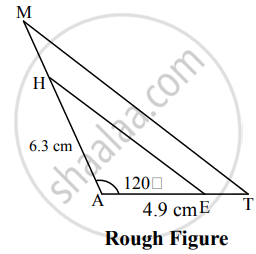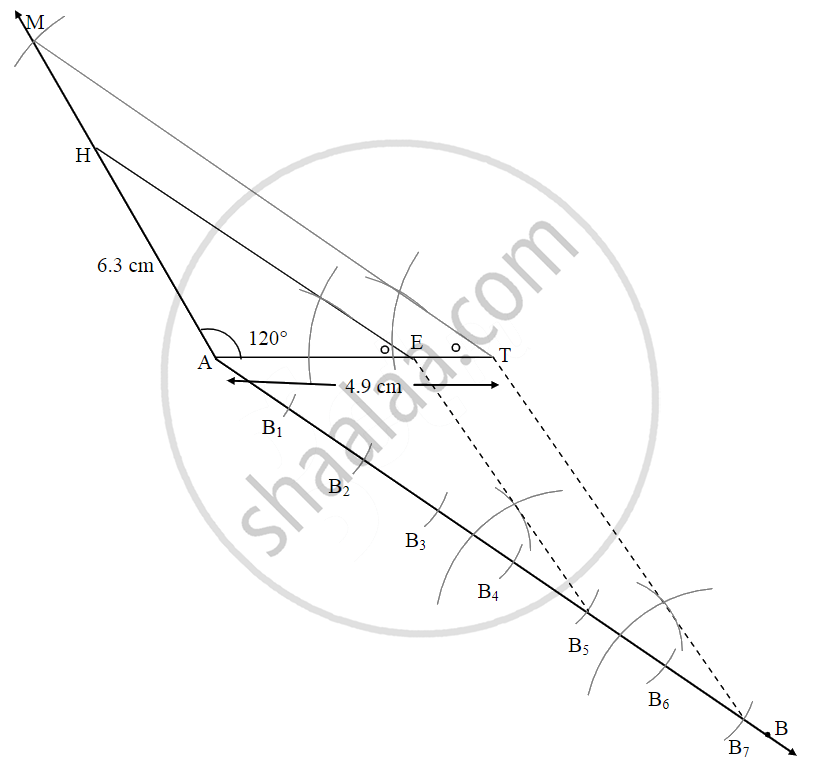# ΔAMT ~ ΔAHE. In ΔAMT, AM = 6.3 cm, ∠MAT = 120°, AT = 4.9 cm, AMHA=75, then construct ΔAMT and ΔAHE - Geometry

Diagram

ΔAMT ~ ΔAHE. In ΔAMT, AM = 6.3 cm, ∠MAT = 120°, AT = 4.9 cm, "AM"/"HA" = 7/5, then construct ΔAMT and ΔAHE

#### SolutionSteps of construction:

1. Draw seg AT of length 4.9 cm
2. Take ∠A as 120° and draw an arc of 6.3 cm on it. Name the point as M.
3. Join seg MT to obtain ΔAMT.
4. Draw ray AB such that ∠TAB is an acute angle.
5. Locate points B1, B2, B3, B4, B5, B6, B7 on ray AB such that,
AB1 = B1B2 = B2B3 = B3B4 = B4B5 = B5B6 = B6B7.
6. Join point T and B7.
7. Through point, B5 draw a line parallel to seg TB7 which intersects seg AT at point E.
8. Draw a line parallel to MT through E to intersect line AM at point H.
∆AHE is the required triangle similar to ∆AMT.
Concept: Division of a Line Segment
Is there an error in this question or solution?

Share